New SAT Math Algebra Practice QuestionsBelow you’ll find some algebra practice questions like the ones you’ll find on the New SAT Math section. Check out these links if you need some background on what “Heart of Algebra” means anyway or how to combine like terms.

SAT Algebra Practice Question 1

Steve and Brian are taking a cross-country road trip. They have agreed to split the cost of gas evenly. The price of gas is \$4 per gallon. If the minivan, when traveling at a constant rate of 60 mph, is able to travel x miles on one gallon, which of the following expressions represents the dollar amount that each pays for a trip covering 150 miles in which the minivan is traveling at a constant rate of 60 mph?

A) 2 (150/x)
B) 4 (x/150)
C) 8 (150/x)
D) 10 (x/150)

SAT Algebra Practice Question 1 Explanation:

The key to getting this question quickly is to figure out how many gallons it will take the minivan to travel 150 miles. Is it (x/150) or (150/x)? You can do a little thought experiment. Imagine that it travels 10 miles on one gallon. How many gallons would it need to travel 150 miles? 150/10 = 15. Therefore, we want (150/x), or either answer choice A) or C). Notice that the two split the cost of gas, which is \$4 per gallon. Therefore, it’s like each is paying \$2 per gallon, or answer A).

If you are struggling to get that, a good idea is to plug in a number for ‘x’ and back-solve. So if we use x = 10, we get 150/10 = 15 gallons at \$4, which equals \$60. The two split that, meaning that each pay \$30. Only A) equals 30.

SAT Algebra Practice Question 2

In the system of equations below, what is the value of x + y?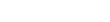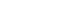A)1/4
B) 19/4
C) 21/4
D) 5

SAT Algebra Practice Question 2 Explanation

We want to make sure, when solving for x and y in an equation that has two variables, that x and y are on the same side of the equation. Then, we multiply either the top or the bottom by a number that will allow us to isolate for either ‘x’ or ‘y’. We then solve for that variable and plug that value back into one of the two equations to find the value of the second variable.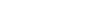This can be simplified to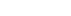Adding the second equation underneath we getAdding the two equations vertically, we get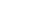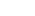Plugging ‘x’ back in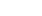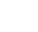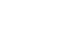, which is (C).

SAT Algebra Practice Question 3

Which of the following lines is not perpendicular to the line with equation 2y + 5x = -11?

A) 5y = 2x + 3/2
B) y = -5/2x + 6
C) 10y – 5 = 4x
D) -5y = -2x -4

SAT Algebra Practice Question 3 Explanation:

Coordinate geometry, when it deals with a linear equation like the one above, is categorized as Heart of Algebra. In this question, we need to find a line that is not perpendicular to 2y + 5x = -11. Perpendicularity is defined as the negative reciprocal of the slope. The slope of that line, once we move things into the y = mx + b form, is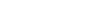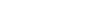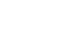Therefore the slope is -5/2. The negative reciprocal, or a line that is perpendicular, would have a slope of 2/5.

All the answer choices have a slope of 2/5 except answer choice B), which has a slope of -5/2. Therefore, answer B).

Author

•Chris Lele is the Principal Curriculum Manager (and vocabulary wizard) at Magoosh. Chris graduated from UCLA with a BA in Psychology and has 20 years of experience in the test prep industry. He's been quoted as a subject expert in many publications, including US News, GMAC, and Business Because. In his time at Magoosh, Chris has taught countless students how to tackle the GRE, GMAT, SAT, ACT, MCAT (CARS), and LSAT exams with confidence. Some of his students have even gone on to get near-perfect scores. You can find Chris on YouTube, LinkedIn, Twitter and Facebook!